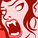# QlikView App Dev

Discussion Board for collaboration related to QlikView App Development.

Announcements
Join this live chat April 6, 10AM EST - QlikView to Qlik Sense REGISTER
cancel
Showing results for
Search instead for
Did you mean:Contributor III

## If and Sum - different results

Hi,

Why do these 2 expressions give different results?     Currency is in a separate table and not associated with other tables.

1:

sum({<Year=,Quarter=,Month=>} if(Currency = ‘Local’, [Local Amount], [Group Amount]))

2:

if(Currency = ‘Local’,

sum({<Year=,Quarter=,Month=>} [Local Amount])

sum({<Year=,Quarter=,Month=>} [Group Amount]))

The first expression gives the correct result - but I like the second expression the best

Regards

Nils

2 RepliesMVP & Luminary

I think you need to use Sum().  Try below expression.

=Sum(if(Currency = ‘Local’,

sum({<Year=,Quarter=,Month=>} [Local Amount])

sum({<Year=,Quarter=,Month=>} [Group Amount])))

Regards,

Jagan.Specialist

My understanding is that when you use sum(if(.... your test and calculation is being run once for each datapoint. However, if you use if(sum(... then you are summing all the datapoints first, and then testing the result. This isn't what is usually required, as in this case where the currency is relevant to each Amount.

I agree that if..sum feels more logical, but after a while sum .. if doesn't seems quite natural too.

Jonathan